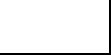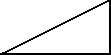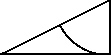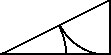#### Graphic construction of the golden sectionDraw a segment and then lead the perpendicular from one end, with a length corresponding to half of the given segment.Join the two ends of the segment to obtain a right triangleWith a compass draw an arc with a radius corresponding to the cathetus of the triangle, until it meets the hypotenuseDraw an arc by pointing the compass at the other vertex at an acute angle, from the point where the first arc cuts the hypotenuse down to the base.
Now the base is divided into two parts which are in a GOLDEN SECTION relationship